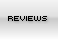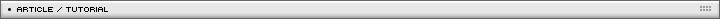Calculating vectors intersection point Page 1/1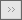[ August 28, 2006 ] Milan Toth (MilGra) An Actionscript 3 article that shows how to calculate vectors intersection points, with source code examples.

A quest for time - calculating vector intersection points

There are several ways to calculate intersection point of vectors, we check the two main ways. The first technique is the per product calculation, the other is the calculation using the function of the line. I was curious which is the most efficient method, because speed does matter.

The per product method: we have two vectors, v1 and v2. We create a third vector between the starting points of these vectors, and calculate the per product of v2 with the two other vectors. Then we have two scalars, and the division of these is the ratio, with which we have to multiply v1 to get the intersection point. It sounds difficult, and it is, that's why i not really like this method :)

v1 ( bx1 , by1 );
v2 ( bx2 , by2 );
v3 ( bx3 , by3 );

n1 ( -by1 , bx1 );
n3 ( -by3 , bx3 );

dp1 = n3.v2 = -by3*bx2 + bx3*by2;
dp2 = n1.v2 = -by1*bx2 + bx1*by2;

rat = dp1/dp2;
crossv = v1*rat;

So we have the intersection point, but we dont know if this point is on both vectors.

The other solution: we create two lines from the vectors, and check for their intersection point.

v1 ( bx1 , by1 );
v2 ( bx2 , by2 );

l1: y1 = m1*x1 + b1;
l2: y2 = m2*x2 + b2;

Where the two lines cross each other:
x1 = x2;
y1 = y2;

so

y = m1*x + b1;
y = m2*x + b2;

making them equal

m1*x + b1 = m2*x + b2;
x*( m1 - m2 ) = b2 - b1;

x = ( b2 - b1 ) / ( m1 - m2 );
y = m1 * x + b1;

And we have the intersection point again. Unfortunately we still don't know if this point is on both vectors. The simplest way to check this is to create new vectors between the intersection point and the endpoints of the original vectors, and check their length, but because this is similar after both methods, we don't use it in our speed test.

Let's see the code. First we need a vector class.

```//begin
//
//
package com.milgra.geometry
{

//
//
//
public class Vector
{
//
//
//
public var bx:Number;
public var by:Number;
public var sx:Number;
public var sy:Number;
public var r:Number;
//
//
//
public function Vector ( stx:Number , sty:Number , enx:Number , eny:Number )
{

//
sx = stx;
sy = sty;
bx = enx - stx;
by = eny - sty;
//
r = Math.sqrt( bx*bx + by*by );
//
}
//
//
//
}
//
//
//
}
//
//
//end
```

I don't implement the vectors as simple direction vectors, i store the starting point, and calculate length, because we may need it in the future.

We need another class, which implements the calculations on the vectors, i called it VectorOperator.

```//begin
//
//
package com.milgra.geometry
{
//
//
//
import flash.utils.*;
import flash.geom.Point;
import com.milgra.geometry.Line;
//
//
//
public class VectorOperator
{
//
//
//
public function VectorOperator ( )
{
//
addLog( "new VectorOperation" );
//
}

//
//
//
public function getPerIntersection ( v1:Vector , v2:Vector ):Point
{
//
//
var v3bx:Number = v2.sx - v1.sx;
var v3by:Number = v2.sy - v1.sy;
var perP1:Number = v3bx*v2.by - v3by*v2.bx;
var perP2:Number = v1.bx*v2.by - v1.by*v2.bx;
var t:Number = perP1/perP2;
//
var cx:Number = v1.sx + v1.bx*t;
var cy:Number = v1.sy + v1.by*t;
//
return new Point( cx , cy );
//
}
```

Nothing spectacular, I just typed down the functions above.

```
//
//
//
public function getLineIntersection ( v1:Vector , v2:Vector ):Point
{
//
//
var m1:Number = v1.by / v1.bx;
var b1:Number = v1.sy - m1*v1.sx;
var m2:Number = v2.by / v2.bx;
var b2:Number = v2.sy - m2*v2.sx;
//
var cx:Number = ( b2 - b1 )/( m1 - m2 );
var cy:Number = m1*cx + b1;
//
return new Point( cx , cy );
//
}

//
//
//
public function addLog( logText:String ):void
{
//
//trace( logText );
//
}
//
//
//
}

//
//
//
}
//
//
//end
```

And we are ready. What we need is a frame program, which creates random vectors, and runs these methods. Download the source, i made a simple vector-drawer class, and i also created a skin using Flash 9 preview to make things more spectacular.

Run it, and press Start button. If you like, you can set vector and crosspoint drawing to true, but watch out, over 5000 cycles drawing freezes your browser. Conclusion:

- running this program on several machines and platforms, the line function method seems 15-20 percent faster, with a few exceptions.
- it worth playing with types in each method, there can be significant speed differencies.

MilGra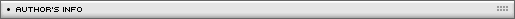Name: Milan Toth (MilGra) Location: Koka, Hungary Age: 24 Flash experience: 4 years Job: flash developer/web designer Website: http://www.tetrapod.ini.hu/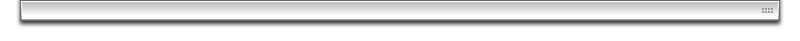| Homepage | News | Games | Articles | Multiplayer Central | Reviews | Spotlight | Forums | Info | Links | Contact us | Advertise | Credits || www.smartfoxserver.com | www.gotoandplay.biz | www.openspace-engine.com | gotoAndPlay() v 3.0.0 -- (c)2003-2008 gotoAndPlay() Team -- P.IVA 03121770048